Calculation of Tesla Coil Output Impedances

I use the MicroSim 8.0 Demo program (free) to calculate Tesla coil impedances.

This is the model I use.  It is here.U1 is a switch that closes at time = 0.
Open resistance = 1e9 ohm.
Closed Resistance = 0.1 ohm.

Rgap is the primary circuit resistance which is mostly the spark gap loss.

Cpri is the Primary capacitance with the initial condition set to the firing voltage (21000 volts in this case).

TC is the Tesla coil with the coupling, primary inductance, and secondary inductance specified.

Csec is the self capacitance and the terminal capacitance combined.

Rload is the streamer power loss resistance.

Cload is the streamer series capacitance which is 1pF per foot.

This model is rather simplified but works for our use here.  The energy is the primary capacitor is either lost in the primary resistance or in the streamer which a very realistic.  The secondary and terminal resistances as well as the charging circuit do not affect the results significantly.

Set the model to run the transient analysis for 8.3333 mS (2 x 60Hz) or 10mS for 50Hz.  I also set the step ceiling for 1uS.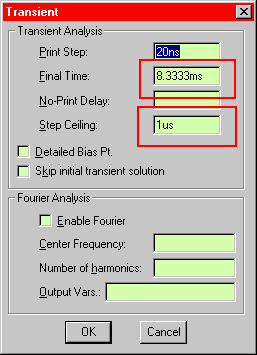Run the model and go to PROBE and you will see the typical voltage and current across Rload the represents the streamer.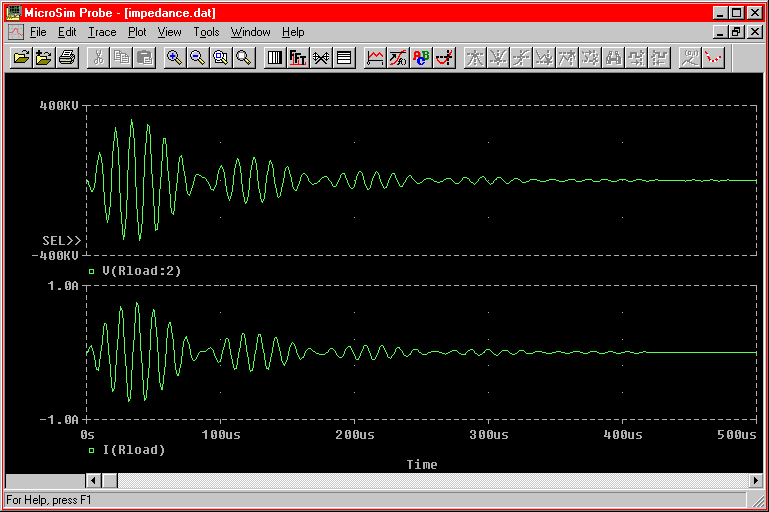In our case, we want to find the RMS voltage and current to the load.  First find the RMS voltage by selecting RMS(V(Rload:2)).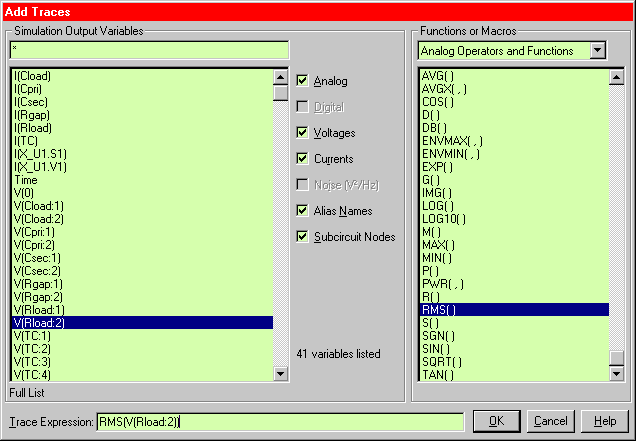Turn on the cursor and go to the right end of the chart.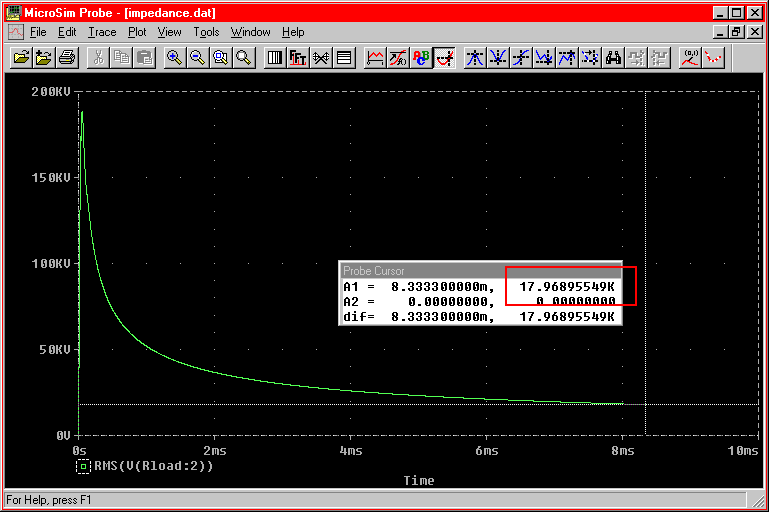This gives the RMS voltage across the load which is 17969 volts RMS.

Now do the same for current by selecting RMS(I(Rload)).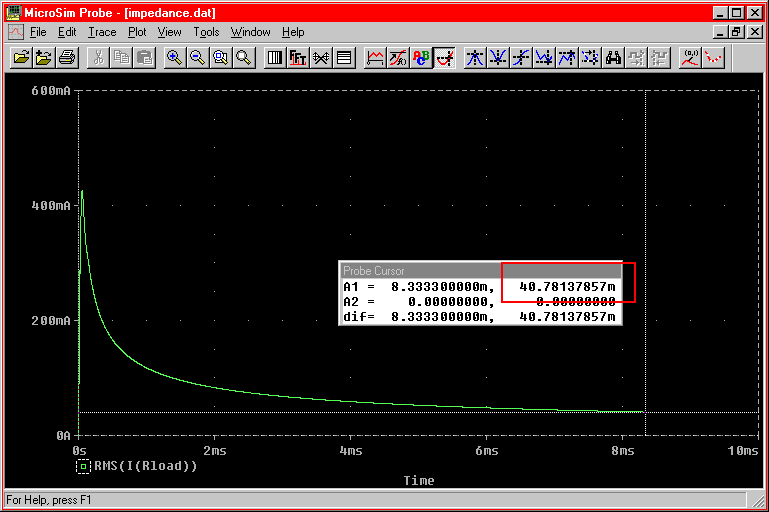This gives the RMS current to the load as 40.78 milliamps.

The LOAD impedance is then calculated by dividing the RMS voltage by the RMS current:

17969 / .04078 = 440632 ohms

It should be noted that this can be calculated by knowing the frequency and impedance of Cload in series with Rload too.  That works out to 426271 ohms.  The difference is due the the primary and secondary circuits being tuned to slightly different frequencies.  The primary is tuned lower to account for streamer loading.

The power the the streamer is simply found with the P = I^2R relation applied to Rload.

(0.04078)^2 x 220000 = 365.86 watts

The input power to the coil is found by finding the energy on the capacitor and multiplying by 120 (or 100 for 50Hz).

E = 1/2 x C x V^2

1/2 x 27.7e-9 x 21000^2 = 6.108 Joules

6.108 x 120 = 733 watts.

Thus the capacitor is delivering 733 watts to the coil and 365.86 watts of that is going to the streamer.  The efficiency happens to be 50%.

Now to find the Tesla coil's output impedance....

First raise the load resistance to 1e9 ohms and short the load series cap.  This effectively makes the coil see an open circuit.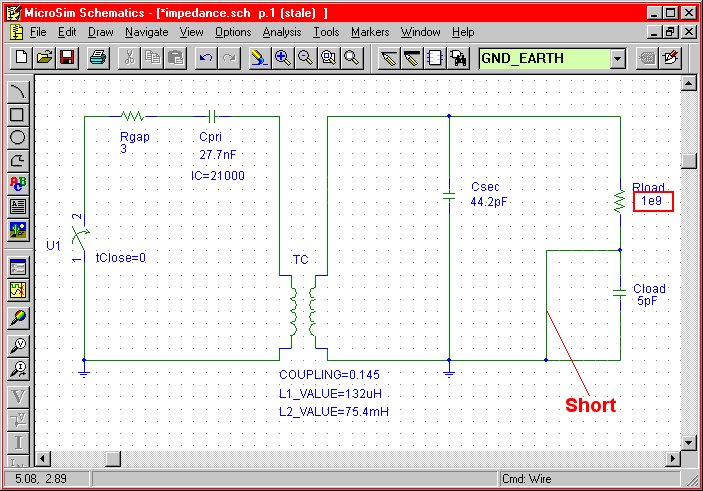Run the models and find the RMS voltage at the terminal.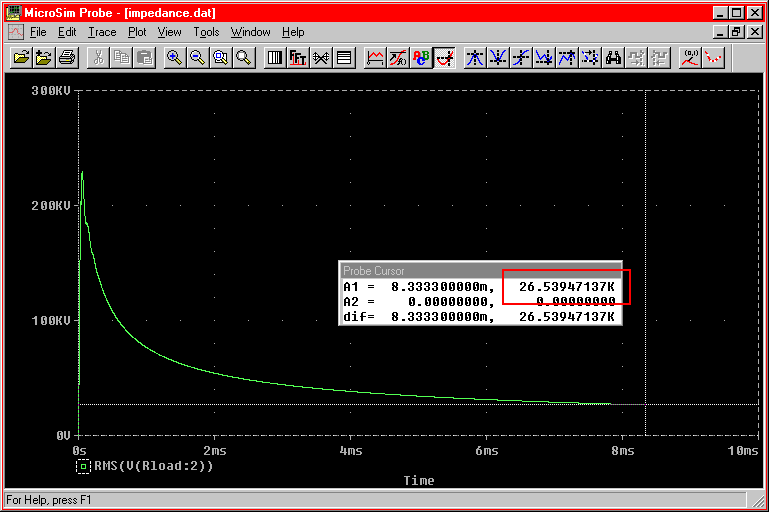We get 26539.5 volts RMS.

Now short the output by reducing the load resistance to 1 ohm.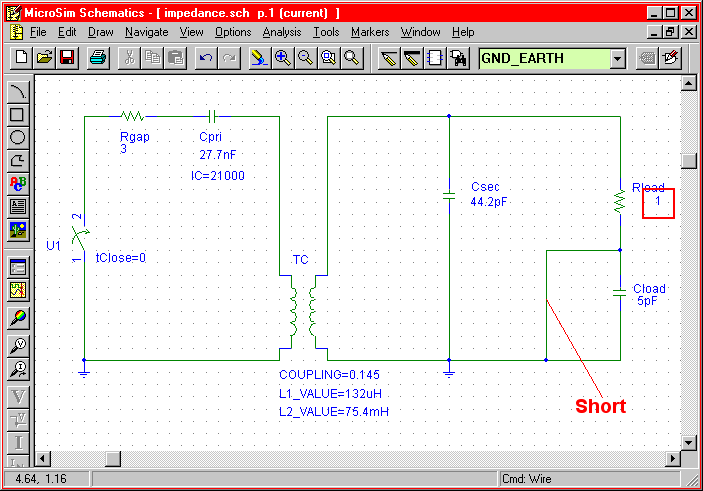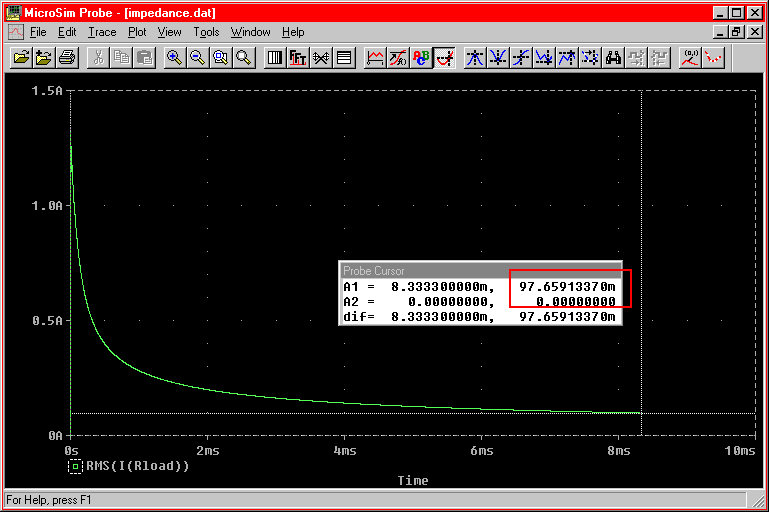We get 97.66 milliamps.

The output impedance is then found by dividing the open circuit voltage by the short circuit current.

26539.5 / 0.09766 = 271754 ohms

That is the basic idea.  The models can be much more complex with more "stuff" added in but the results don't change that much.  I often use the full TC model but it is much slower.  One can find the effects of coupling, primary loss, etc.  I have found the system works best with the output impedance close to the load impedance.  Obviously much more work to be done with all this...

Cheers,

Terry

This site hosted by.....
Tesla Coil Info Depot